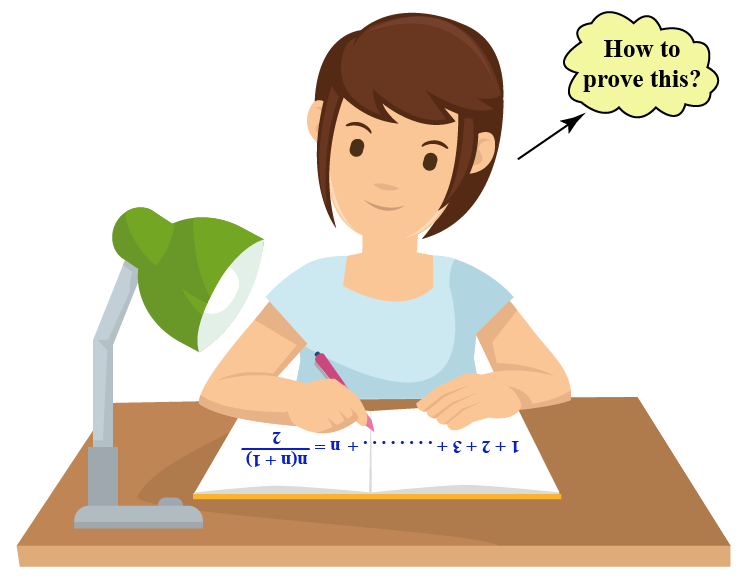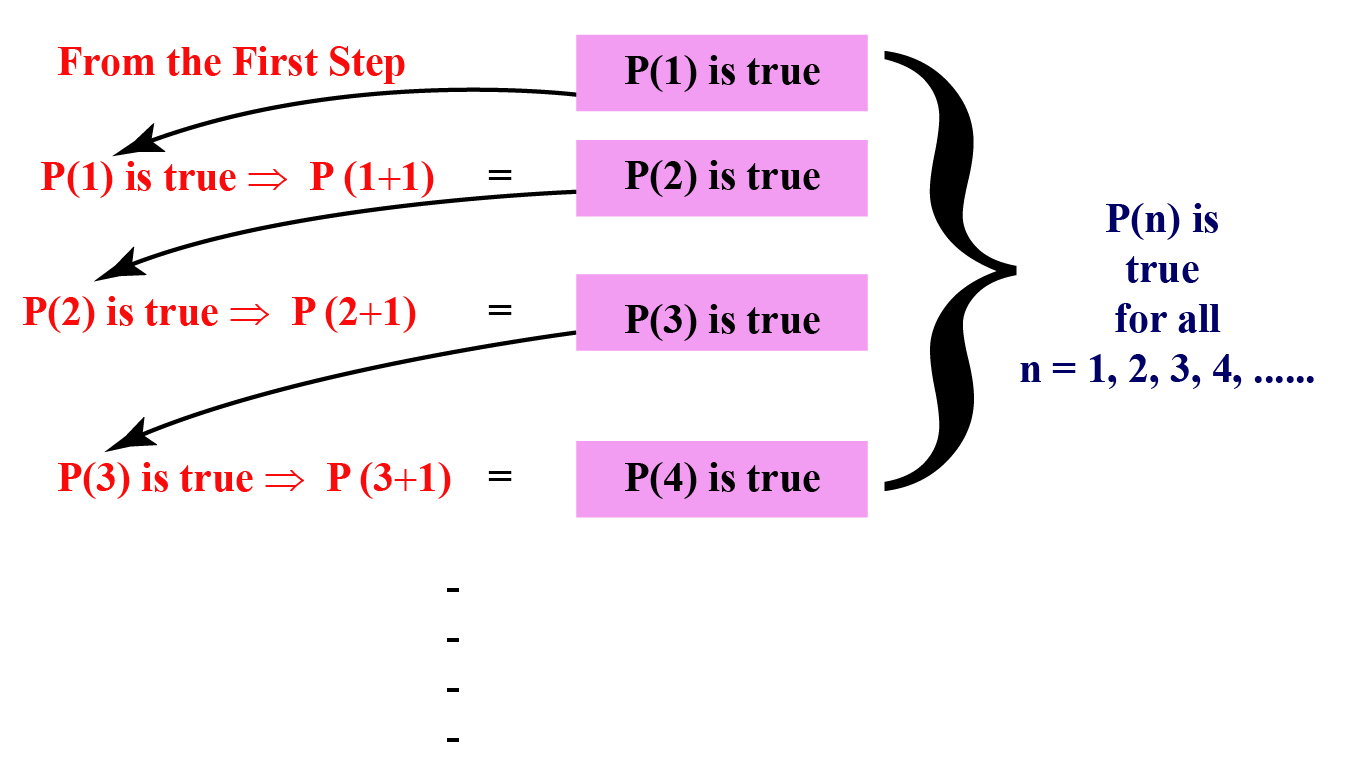# Mathematical Induction

Mathematical Induction

Mia was asked to find the sum of the first 100 natural numbers. She was able to recall this formula:

$1+2+3+...+n = \dfrac{n(n+1)}{2}$

She substituted $$n=100$$ in this formula and got the answer as 5,050

Is this answer correct?

How can you prove this formula?In this lesson, you will learn all about mathematical induction and learn how to prove this formula. You will learn about the principle of mathematical induction and will go through various mathematical induction examples.

Let's begin!

## Lesson Plan

 1 What Is Mathematical Induction? 2 Tips and Tricks on Mathematical Induction 3 Solved Examples 4 Challenging Questions on Mathematical Induction 5 Interactive Questions

## What Is Mathematical Induction?

Mathematical induction is the process of proving a mathematical statement (or) an equation (or) a theorem for the set of all natural numbers, $$N$$.

### Principle of Mathematical Induction

The principle of mathematical induction works in the following way.

To show something works all the time:

1. We have to show that it works for the first time.
2. We should assume it works for this time.
3. Prove that it works for the next time.

From the above steps, we can then say that it works every time.

This is the principle of mathematical induction in words.

We will see the principle of mathematical induction in mathematical terms in the following section.

## How to Prove Mathematical Induction?

Using the principle of mathematical induction, to prove a statement $$P(n)$$ is true for all $$n\in N$$, we have to prove:

1. $$P(1)$$ is true.
2. Assume $$P(k)$$ is true for some $$k \in N$$.
3. Prove $$P(k+1)$$ is true.

Here is the proof of the principle of mathematical induction.## What Are the Steps in Mathematical Induction?

In the previous section, we have seen the steps to prove a statement by mathematical induction.

Each step is named as follows.

1. Base step: To prove $$P(1)$$ is true.
2. Assumption step: Assume that $$P(k)$$ is true for some $$k \in N$$.
3. Induction step: Prove that $$P(k+1)$$ is true.

After proving these 3 steps, we can say that "By the principle of mathematical induction, $$P(n)$$ is true for all $$n \in N$$."

Example

Prove the following by mathematical induction:

$1+2+3+...+n = \dfrac{n(n+1)}{2}$

Solution

Let us assume that $P(n):\,\,1+2+3+...+n = \dfrac{n(n+1)}{2}$

We use the above 3 steps to prove $$P(n)$$.

Base step: Let us prove $$P(n)$$ for $$n=1$$.

\begin{array}{l} \text { LHS = First term = } 1 \\[0.2cm] \begin{aligned} \text{RHS} &=\frac{1(1+1)}{2} \\[0.2cm] &=\frac{1(2)}{2} \\[0.2cm] &=1 \end{aligned} \\[0.2cm] \therefore \quad \text {LHS} = \text {RHS} \end{array}

Thus, $$P(n)$$ is true for $$n=1$$

Assumption step: Assume that $$P(k)$$ is true for some $$k \in N$$.

Thus,

\begin{align} 1+2+3+...+k &= \dfrac{k(k+1)}{2}\,\,\, \rightarrow (1) \end{align}

Induction step: Let us prove $$P(k+1)$$ is true.

i.e., we have to prove:

\begin{align} 1+2+3+&...+(k+1)\0.2cm] &= \dfrac{(k+1)[(k+1)+1]}{2}\end{align} \[ \begin{aligned} \text{LHS} &=1+2+\cdots+(k+1) \\[0.2cm] &=\underbrace{1+2+\cdots+k}+(k+1) \\[0.2cm] &=\frac{k(k+1)}{2}+k+1 \quad(\text { From }(1)) \\[0.2cm] &=\frac{k(k+1)}{2}+\frac{2(k+1)}{2}\\[0.2cm] &=\frac{k^{2}+k+2 k+2}{2}\\[0.2cm] &=\frac{k^{2}+3 k+2}{2}\\[0.2cm] &=\frac{(k+1)(k+2)}{2}\\[0.2cm] &=\frac{(k+1)((k+1)+1)}{2}\\[0.2cm] &=\text{RHS} \end{aligned}

Thus, $$P(n)$$ is true for $$n=k+1$$

Therefore, by the principle of mathematical induction, $$P(n)$$ is true for all $$n \in N$$.Tips and Tricks

The "induction step" is the most difficult part of the "proof by mathematical induction" method. Here are some tips to get through it:

1. $$k^{th}$$ term comes just before $$(k+1)^{th}$$ term.
2. The result of the "assumption step" is used after writing the $$k^{th}$$ term (before the $$(k+1)^{th}$$ term).
3. If getting the RHS from the LHS seems difficult, simplify the LHS and the RHS separately and prove that they are equal.

## Solved Examples

Here are a few mathematical induction examples.

 Example 1

Henry is asked to prove the following by the principle of mathematical induction for all $$n \in N$$. Can we help him?

\begin{align}1^{2}+3^{2}+5^{2}+&\ldots+(2 n-1)^{2}\\[0.2cm]&=\frac{n(2 n-1)(2 n+1)}{3}\end{align}

Solution

Let us assume the given equation to be $$P(n)$$.

Base step: Let us prove $$P(n)$$ for $$n=1$$

\begin{array}{l} \text { LHS = First term = } 1^2=1 \\[0.2cm] \begin{aligned} \text{RHS} &=\frac{1(2-1)(2+1)}{3} \\[0.2cm] &=\frac{1(3)}{3} \\[0.2cm] &=1 \end{aligned} \\[0.2cm] \therefore \quad \text {LHS} = \text {RHS} \end{array}

Thus, $$P(n)$$ is true for $$n=1$$

Assumption step: Assume that $$P(k)$$ is true for some $$k \in N$$. Then we get:

\begin{align}1^{2}+&3^{2}+5^{2}+\ldots+(2 k-1)^{2}\\[0.2cm]&=\frac{k(2 k-1)(2 k+1)}{3} \,\, \rightarrow (1) \end{align}

Induction step: Let us prove $$P(k+1)$$ is true.

i.e., we have to prove:

\begin{align}&1^{2}+3^{2}+5^{2}+\ldots+[2 (k+1)-1]^{2}\0.2cm]&=\dfrac{(k+1)[2(k+1)-1][2(k+1)+1]}{3}\end{align} \begin{aligned} &\text{LHS}\\[0.2cm] &=1^{2}+3^{2}+5^{2}+\ldots+[2(k+1)-1]^{2}\\[0.2cm] &=\underbrace{1^{2}+3^{2}+5^{2}+\ldots+(2 k-1)^{2}}+[2 (k+1)-1]^{2}\\[0.2cm] &=\frac{k(2 k-1)(2 k+1)}{3}+(2 k+1)^{2} \,[\text { from (1)} ]\\[0.2cm] &=\frac{k(2 k-1)(2 k+1)+3(2 k+1)^{2}}{3}\\[0.2cm] &=\frac{(2 k+1)[k(2 k-1)+3(2 k+1)]}{3}\\[0.2cm] &=\frac{(2 k+1)\left[2 k^{2}-k+6 k+3\right]}{3}\\[0.2cm] &=\frac{(2 k+1)\left[2 k^{2}+5 k+3\right]}{3}\\[0.2cm] &=\frac{(2 k+1)\left[2 k^{2}+2 k+3 k+3\right]}{3}\\[0.2cm] &=\frac{(2 k+1)[2 k(k+1)+3(k+1)]}{3}\\[0.2cm] &=\frac{(2 k+1)(k+1)(2 k+3)}{3}\\[0.2cm] &=\frac{(k+1)[2(k+1)-1][2(k+1)+1]}{3}\\[0.2cm] &=\text{RHS} \end{aligned} Hence, $$P(n)$$ is true for $$n=k+1$$ Thus, by the principle of mathematical induction, $$P(n)$$ is true for all $$n \in N$$.  $$\therefore$$ Hence proved  Example 2 Can we help Jake prove the following by the principle of mathematical induction for all $$n \in N$$? \[\begin{align}a+a r+a r^{2}&+\ldots+a r^{n-1}\\[0.2cm]&=\frac{a\left(r^{n}-1\right)}{r-1}\end{align}

Solution

Let us assume the given equation to be $$P(n)$$.

Base step: Let us prove $$P(n)$$ for $$n=1$$.

\begin{array}{l} \text { LHS =First term = } a \\[0.2cm] \begin{aligned} \text{RHS} &=\frac{a\left(r-1\right)}{r-1} \\[0.2cm] &=a \end{aligned} \\[0.2cm] \therefore \quad \text {LHS} = \text {RHS} \end{array}

So $$P(n)$$ is true for $$n=1$$.

Assummtion step: Assume that $$P(k)$$ is true for some $$k \in N$$. Then we get:

\begin{aligned}a+a r&+a r^{2}+\ldots+a r^{k-1}\\[0.2cm]&=\frac{a\left(r^{k}-1\right)}{r-1}\,\rightarrow(1) \end{aligned}

Induction step: Let us prove $$P(k+1)$$ is true.

i.e., we have to prove:

\begin{align}a+a r&+a r^{2}+\ldots+a r^{(k+1)-1}\0.2cm]& =\frac{a\left(r^{k+1}-1\right)}{r-1}\end{align} \begin{aligned} &\text{LHS}\\[0.2cm] &=a+a r+a r^{2}+\ldots+a r^{(k+1)-1}\\[0.2cm] &=\underbrace{\left[a+a r+a r^{2}+\ldots+a r^{k-1}\right]}+a r^{(k+1)-1}\\[0.2cm] &=\frac{a\left(r^{k}-1\right)}{r-1}+a r^{k} \,\,\,[\text { from (1)} ]\\[0.2cm] &=\frac{a\left(r^{k}-1\right)+a r^{k}(r-1)}{r-1}\\[0.2cm] &=\frac{a r^{k}-a+a r^{k+1}-a r^{k}}{r-1}\\[0.2cm] &=\frac{a r^{k+1}-a}{r-1}\\[0.2cm] &=\frac{a\left(r^{k+1}-1\right)}{r-1}\\[0.2cm] &=\text{RHS} \end{aligned} Hence, $$P(n)$$ is true for $$n=k+1$$. Thus, by the principle of mathematical induction, $$P(n)$$ is true for all $$n \in N$$.  $$\therefore$$ Hence proved.  Example 3 Can we help John prove the following by the principle of mathematical induction: $$10^{2 n-1}+1$$ is divisible by 11 Solution Let us assume the given statement to be $$P(n)$$. Base step: When $$n = 1$$, $$10^{2 (1)-1}+1 =11$$, which is divisible by 11 Hence, $$P(n)$$ is true for $$n=1$$ Assumption step: Assume that $$P(k)$$ is true for some $$k \in N$$. We get: $$10^{2 k-1}+1$$ is divisible by 11 We can write $$10^{2 k-1}+1$$ as a multiple of 11 \[10^{2 k-1}+1 = 11a \,\,\,\, \rightarrow (1)

Here, $$a$$ is any integer.

Induction step: Let us prove $$P(k+1)$$ is true. For this consider,

\begin{aligned} &10^{2(k+1)-1}+1 \\[0.2cm] &=10^{2k+2-1}+1 \\[0.2cm] &=10^{2 k+1}+1 \\[0.2cm] &=10^{2}\left(10^{2 k-1}\right)+1 \\[0.2cm] &=10^{2}\left(10^{2 k-1}+1-1\right)+1 \\[0.2cm] &=10^{2}\underbrace{\left(10^{2 k-1}+1\right)}-10^{2}+1 \\[0.2cm] &=10^{2} \cdot 11 a-100+1 \,\,\, \text{ [ From (1)] } \\[0.2cm] &=10^{2} \cdot 11 a-99 \\[0.2cm] &=11(100 a-9) \end{aligned}

So $$10^{2(k+1)-1}+1$$ is divisible by 11 (as it is a multiple of 11)

So $$P(n)$$ is true for $$n=k+1$$

Thus, by the principle of mathematical induction, $$P(n)$$ is true for all $$n \in N$$.

 $$\therefore$$ Hence provedChallenging Questions

Prove the following by the principle of mathematical induction:

1. $$41^{n}-14^{n}$$ is a multiple of 27
2. $$(2 n+7)<(n+3)^{2}$$.

## Interactive Questions

Here are a few activities for you to practice.

Select/type your answer and click the "Check Answer" button to see the result.

## Let's Summarize

We hope you enjoyed learning about Mathematical Induction with the simulations and practice questions. Now, you will be able to easily solve problems on the principle of mathematical induction and mathematical induction examples.

## About Cuemath

At Cuemath, our team of math experts are dedicated to make learning fun for our favorite readers, the students! Through an interactive and engaging learning-teaching-learning approach, the teachers explore all angles of a topic. Be it problems, online classes, videos, or any other form of relation, it’s the logical thinking and smart learning approach that we at Cuemath believe in.

## 1. When can you use mathematical induction?

We use mathematical induction to prove a mathematical equation, statement, (or) a theorem.

## 2. Why is mathematical induction important?

Mathematical induction is important because we can use it to prove a mathematical equation, statement, (or) a theorem.

## 3. What is the use of mathematical induction in real life?

Recall the sinking of the Titanic. The crew of the Titanic realized that when a given bulkhead was completely flooded, the next bulkhead would undergo the same fate, thus sinking the whole ship.

More Important Topics
Numbers
Algebra
Geometry
Measurement
Money
Data
Trigonometry
Calculus